• 机器学习中的逻辑回归和线性回归的matlab程序实现 一、逻辑回归(用于分类) 1、逻辑回归样本数据集的生成程序： clear all; a = input('please input weights matrix:\n'); [row,col] =size(a); num = input...
机器学习中的逻辑回归和线性回归的matlab程序实现
一、逻辑回归(用于分类)
1、逻辑回归样本数据集的生成程序：
clear all;
a = input('please input weights matrix:\n');
[row,col] =size(a);

num = input('how many numbers do you want"\n');
class = zeros(num,1);
rand_input = roundn(10*rand(num,col-1),-2);
rand_output = roundn(21*rand(num,1),-2);
output = roundn(rand_input(1:num,1:col-1)*a(1,2:col)' + ones(num,1)*a(1,1)+ wgn(num,1,0),-2);
for i = 1:num
if rand_output(i,1) > output(i,1)
class(i,1) = 1;
else
class(i,1) = 0;
end
end
txt_data = [rand_input,rand_output,class];

data_base = fopen('Ldata.txt','w');
for j = 1:num
for i = 1:col+1
fprintf(data_base,'%4.2f\t',txt_data(j,i));
end
fprintf(data_base,'\n');
end

使用说明：  （1）由于在实现算法中使用的二维平面图来显示逻辑回归的分类效果，所以只能生成输入权重为2的测试矩阵，即一元一阶函数，如[5 2]，y=2x+5（当然，也可以用于多元一阶函数；  （2）输入要生成的样本总数，样本数量越大，分类效果越好，但是处理时间越长。

例如：权重矩阵为[10 0.5],样本数量为5000，对应的二维图显示如下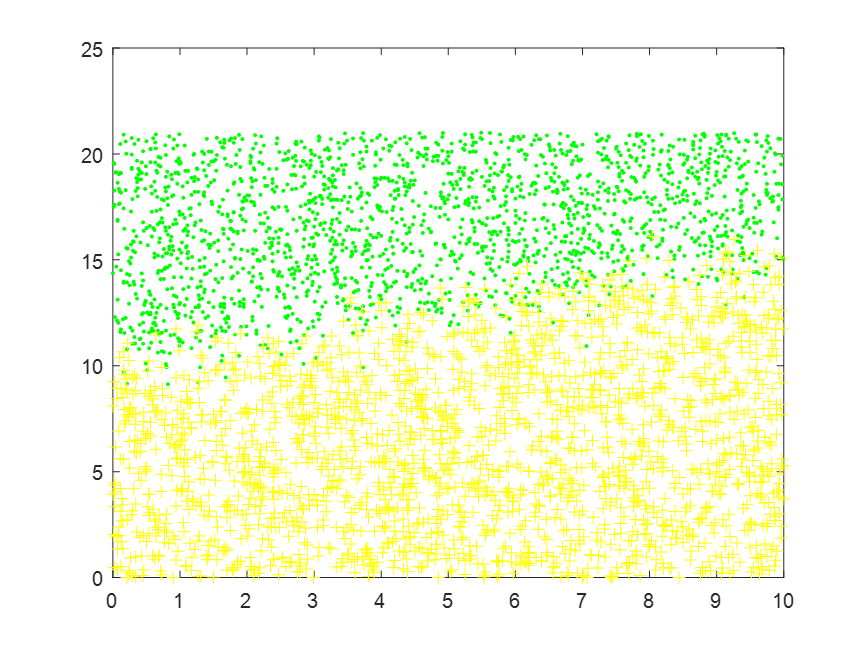2、用逻辑回归实现分类的程序
clear all;
%从文本文件输入数据
ex = importdata('Ldata.txt');
[row,col] = size(ex);
%逻辑回归固定两个特征
class_1 = zeros(row,2);
class_2 = zeros(row,2);
i = 0;
j = 0;
%绘制二维图
for num = 1:row
if(ex(num,3) == 1)
i = i+1;
class_1(i,:) = [ex(num,1),ex(num,2)];
else
j = j+1;
class_2(j,:) = [ex(num,1),ex(num,2)];
end
end
plot(class_1(1:i,1),class_1(1:i,2),'g.',class_2(1:i,1),class_2(1:i,2),'y+');
hold on;

%定义特征数，样本数,最后一列为输出
feature = col;
example = row;
%定义权重矩阵,输入矩阵,输出矩阵
weight = ones(example,feature);
input_x = [ones(example,1),ex(1:example,1:feature - 1)];
output_y = ex(:,col);
%d定义预测值矩阵，误差矩阵
predict = zeros(example,1);
feature_err = zeros(example,1);

%确定学习率
learning_rate = input('please input rate of learning:\n');
sum = 0;
%梯度下降
for num = 1:example
%如果遍历到最后一个样本，则跳出
if num == example
break;
end
%遍历每一个权重
for j = 1:feature
sum = 0;
%当前所遍历到的样本求和
for time = 1:num
%用上一次权重求预测值
%===========使用sigmod函数=======================
predict(time) = 1/(exp(-input_x(time,:) * weight(num,:)') + 1);
sum = sum + (predict(time) - output_y(time)) * input_x(time,j);
end
weight(num + 1,j) = weight(num,j) - learning_rate/num*sum;
end
%求前后两次特征误差
feature_err(num) = (weight(num+1,:) - weight(num,:))*(weight(num+1,:) - weight(num,:))';
if feature_err(num) < 0.000001
break;
end
end
x1 = 0:10;
x2 =-(weight(time,2)*x1 + weight(time,1))/weight(time,3);
plot(x1,x2,'r-');
hold off;
使用说明：  (1) 该程序使用的样本即为以上程序所生成的。  (2) 学习率，即梯度下降的步长需要手动调节，目前还没有自动调节的功能。

例如：  使用上例产生的[10 0.5]*5000的样本数据，设置学习率a=0.2（手动调节所得出的最佳学习率），达到收敛的迭代次数为2376次，分类效果对应的二维图显示如下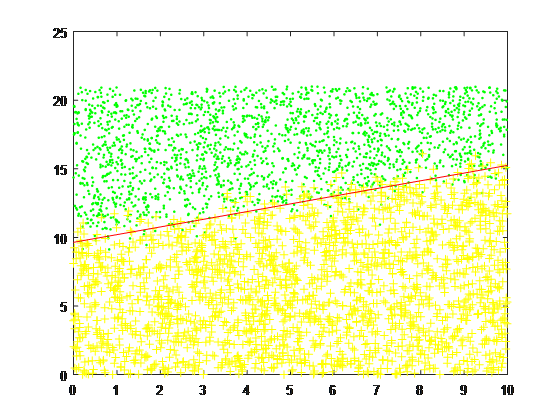二、线性回归（用于拟合）
1、线性回归样本数据集的生成程序：
clear all;
a = input('please input weights martix:\n');
[row,col] =size(a);

num = input('how many numbers do you want"\n');
rand_input = roundn(10*rand(num,col-1),-2);
rand_output = roundn(rand_input*a(1,2:col)' + ones(num,1)*a(1,1) + wgn(num,1,0),-2);

txt_data = [rand_input,rand_output];

data_base = fopen('data.txt','w');
for j = 1:num
for i = 1:col
fprintf(data_base,'%f\t',txt_data(j,i));
end
fprintf(data_base,'\n');
end
说明：  (1)输入权重矩阵可以为单行多列，即拟合的目标函数可为多元一次函数，例如[1 2 3 4 5],y=1 + 2x1 + 3x2 + 4x3 +5x4  (2)可选择样本集的数量。

例如：权重矩阵为[1 2 3 4 5],样本数量为1000，部分数据如下
X1X2X3X4Y6.3200001.6900000.4600007.85000058.7900009.8500009.5200008.8500001.31000089.9200005.5900005.4300008.4000000.51000061.9600009.3400002.5100001.1800006.28000065.4600007.2000005.7900004.1000000.29000049.8900004.8400009.1500001.2000001.36000050.5700006.3900008.9600005.7200006.95000098.3100008.8800004.8300009.4900005.16000096.0200001.9900004.4300002.5600005.43000055.620000

2、用线性回归实现拟合的程序
clear all;
%从文本文件输入数据
ex = importdata('data.txt');
[row,col] = size(ex);
%定义特征数，样本数,最后一列为输出
feature = col;
example = row;
%定义权重矩阵,输入矩阵,输出矩阵
weight = ones(example,feature);
input_x = [ones(example,1),ex(1:example,1:feature - 1)];
output_y = ex(:,col);
%d定义预测值矩阵，误差矩阵
predict = zeros(example,1);
feature_err = zeros(example,1);

%确定学习率
learning_rate = input('please input rate of learning:\n');
sum = 0;
%梯度下降
for num = 1:example
%如果遍历到最后一个样本，则跳出
if num == example
break;
end
%遍历每一个权重
for j = 1:feature
sum = 0;
%当前所遍历到的样本求和
for time = 1:num
%用上一次权重求预测值
predict(time) = input_x(time,:) * weight(num,:)';
sum = sum + (predict(time) - output_y(time)) * input_x(time,j);
end
weight(num + 1,j) = weight(num,j) - learning_rate/num*sum;
end
%求前后两次特征误差
feature_err(num) = (weight(num+1,:) - weight(num,:))*(weight(num+1,:) - weight(num,:))';
if feature_err(num)<0.000000001
break;
end
end
说明：  (1) 使用的样本集即为上一程序所生成。  (2) 学习率需自行设置、调试并取其中效果最好。

例如：  使用上例产生的[1 2 3 4 5]*5000的样本数据，设置学习率a=0.01  达到收敛的迭代次数为3228次。  从3224至3229的拟合效果如下：
bX1X2X3X41.042717205529341.995659261199243.005272512806473.998613417585194.990207805105081.042689158246761.995677642067953.005273439661663.998610112634264.990205971868301.042660097631201.995689928092603.00526622462293.998604203182704.990202032782601.042631532417371.995703428163713.005262198588683.998601155746954.990200710248441.042604412571701.995720506677453.005266175651683.998611280001134.990211908643721.042575075316811.995724077556263.005256751030333.998608856659034.99020939145987
可见，拟合效果尚且可行。
三、总结
以上两类程序均是基于梯度下降算法完成，其中逻辑回归算法的代价函数使用的是sigmod函数。
展开全文机器学习 matlab
• 一劳永逸的回归和分类。 该存储库包含四个文件： Leave_one_out_analysis.m：用于简单的留一法分析的MATLAB代码。 manual.docx：与该页面的内容基本相同。 loo_regression_data.xlsx：用于回归的示例电子表格。 loo_...
• InsuLearn是一个直观而强大的分布式系统，旨在对医疗数据进行回归和分类，同时保留数据的安全性隐私性。 您可以阅读描述该项目的已发表论文： 。 Alborz Amir-Khalili，Soheil Kianzad，Rafeef Abugharbieh，Ivan ...
• 回归分类的区别在于：回归所预测的目标量的取值是连续的（例如房屋的价格）；而分类所预测的目标变量的取值是离散的（例如判断邮件是否为垃圾邮件）。当然，为了便于理解，我们从二值分类（binary classification...

逻辑回归原理及实现

虽然叫做“回归”，但是这个算法是用来解决分类问题的。回归与分类的区别在于：回归所预测的目标量的取值是连续的（例如房屋的价格）；而分类所预测的目标变量的取值是离散的（例如判断邮件是否为垃圾邮件）。当然，为了便于理解，我们从二值分类（binary classification）开始，在这类分类问题中，y只能取0或1。更好的理解问题，先举个小例子：假如我们要制作一个垃圾邮件过滤系统，如果一封邮件是垃圾系统，y=1，否则y=0 。给定训练样本集，当然它们的特征和label都已知，我们就是要训练一个分类器，将它们分开。
回归分析用来描述自变量x和因变量Y之间的关系，或者说自变量X对因变量Y的影响程度，并对因变量Y进行预测。其中因变量是我们希望获得的结果，自变量是影响结果的潜在因素，自变量可以有一个，也可以有多个。一个自变量的叫做一元回归分析，超过一个自变量的叫做多元回归分析。下面是一组广告费用和曝光次数的数据，费用和曝光次数一一对应。其中曝光次数是我们希望知道的结果，费用是影响曝光次数的因素，我们将费用设置为自变量X，将曝光次数设置为因变量Y，通过一元线性回归方程和判定系数可以发现费用(X)对曝光次数(Y)的影响。
1、 逻辑回归模型
回归是一种极易理解的模型，就相当于y=f(x)，表明自变量x与因变量y的关系。最常见问题有如医生治病时的望、闻、问、切，之后判定病人是否生病或生了什么病，其中的望闻问切就是获取自变量x，即特征数据，判断是否生病就相当于获取因变量y，即预测分类。
最简单的回归是线性回归，在此借用Andrew NG的讲义，有如图1.a所示，X为数据点——肿瘤的大小，Y为观测值——是否是恶性肿瘤。通过构建线性回归模型，如hθ(x)所示，构建线性回归模型后，即可以根据肿瘤大小，预测是否为恶性肿瘤hθ(x)≥.05为恶性，hθ(x)<0.5为良性。图1 线性回归示例
然而线性回归的鲁棒性很差，例如在图1.b的数据集上建立回归，因最右边噪点的存在，使回归模型在训练集上表现都很差。这主要是由于线性回归在整个实数域内敏感度一致，而分类范围，需要在[0,1]。逻辑回归就是一种减小预测范围，将预测值限定为[0,1]间的一种回归模型，其回归方程与回归曲线如图2所示。逻辑曲线在z=0时，十分敏感，在z>>0或z<<0处，都不敏感，将预测值限定为(0,1)。图2 逻辑方程与逻辑曲线
逻辑回归其实仅为在线性回归的基础上，套用了一个逻辑函数，但也就由于这个逻辑函数，逻辑回归成为了机器学习领域一颗耀眼的明星，更是计算广告学的核心。对于多元逻辑回归，可用如下公式似合分类，其中公式(4)的变换，将在逻辑回归模型参数估计时，化简公式带来很多益处，y={0,1}为分类结果。对于训练数据集，特征数据x={x1, x2, … , xm}和对应的分类数据y={y1, y2, … , ym}。构建逻辑回归模型f(θ)，最典型的构建方法便是应用极大似然估计。首先，对于单个样本，其后验概率为：那么，极大似然函数为：log似然是：2、 梯度下降
由第1节可知，求逻辑回归模型f(θ)，等价于：采用梯度下降法：从而迭代θ至收敛即可：2.1 梯度下降算法
梯度下降算法的伪代码如下：
################################################
初始化回归系数为1
重复下面步骤直到收敛{
计算整个数据集的梯度
}
返回回归系数值
################################################
注：因为本文中是求解的Logit回归的代价函数是似然函数，需要最大化似然函数。所以我们要用的是梯度上升算法。但因为其和梯度下降的原理是一样的，只是一个是找最大值，一个是找最小值。找最大值的方向就是梯度的方向，最小值的方向就是梯度的负方向。不影响我们的说明，所以当时自己就忘了改过来了，谢谢评论下面@wxltt的指出。另外，最大似然可以通过取负对数，转化为求最小值。代码里面的注释也是有误的，写的代码是梯度上升，注销成了梯度下降，对大家造成的不便，希望大家海涵。

梯度下降算法在每次更新回归系数的时候都需要遍历整个数据集（计算整个数据集的回归误差），该方法对小数据集尚可。但当遇到有数十亿样本和成千上万的特征时，就有点力不从心了，它的计算复杂度太高。改进的方法是一次仅用一个样本点（的回归误差）来更新回归系数。这个方法叫随机梯度下降算法。由于可以在新的样本到来的时候对分类器进行增量的更新（假设我们已经在数据库A上训练好一个分类器h了，那新来一个样本x。对非增量学习算法来说，我们需要把x和数据库A混在一起，组成新的数据库B，再重新训练新的分类器。但对增量学习算法，我们只需要用新样本x来更新已有分类器h的参数即可），所以它属于在线学习算法。与在线学习相对应，一次处理整个数据集的叫“批处理”。
随机梯度下降算法的伪代码如下：
###############################################
初始化回归系数为1
重复下面步骤直到收敛{
对数据集中每个样本
计算该样本的梯度
}
返回回归系数值
###############################################

2.3 改进的随机梯度下降
1）在每次迭代时，调整更新步长alpha的值。随着迭代的进行，alpha越来越小，这会缓解系数的高频波动（也就是每次迭代系数改变得太大，跳的跨度太大）。当然了，为了避免alpha随着迭代不断减小到接近于0（这时候，系数几乎没有调整，那么迭代也没有意义了），我们约束alpha一定大于一个稍微大点的常数项，具体见代码。
2）每次迭代，改变样本的优化顺序。也就是随机选择样本来更新回归系数。这样做可以减少周期性的波动，因为样本顺序的改变，使得每次迭代不再形成周期性。
改进的随机梯度下降算法的伪代码如下：
################################################
初始化回归系数为1
重复下面步骤直到收敛{
对随机遍历的数据集中的每个样本
随着迭代的逐渐进行，减小alpha的值
计算该样本的梯度
}
返回回归系数值
#################################################

3、Python实现
训练数据：1 -0.017612    14.053064    0
2 -1.395634    4.662541    1
3 -0.752157    6.538620    0
4 -1.322371    7.152853    0
5 0.423363    11.054677    0
6 0.406704    7.067335    1
7 0.667394    12.741452    0
8 -2.460150    6.866805    1
9 0.569411    9.548755    0
10 -0.026632    10.427743    0
11 0.850433    6.920334    1
12 1.347183    13.175500    0
13 1.176813    3.167020    1
14 -1.781871    9.097953    0
15 -0.566606    5.749003    1
16 0.931635    1.589505    1
17 -0.024205    6.151823    1
18 -0.036453    2.690988    1
19 -0.196949    0.444165    1
20 1.014459    5.754399    1
21 1.985298    3.230619    1
22 -1.693453    -0.557540    1
23 -0.576525    11.778922    0
24 -0.346811    -1.678730    1
25 -2.124484    2.672471    1
26 1.217916    9.597015    0
27 -0.733928    9.098687    0
28 -3.642001    -1.618087    1
29 0.315985    3.523953    1
30 1.416614    9.619232    0
31 -0.386323    3.989286    1
32 0.556921    8.294984    1
33 1.224863    11.587360    0
34 -1.347803    -2.406051    1
35 1.196604    4.951851    1
36 0.275221    9.543647    0
37 0.470575    9.332488    0
38 -1.889567    9.542662    0
39 -1.527893    12.150579    0
40 -1.185247    11.309318    0
41 -0.445678    3.297303    1
42 1.042222    6.105155    1
43 -0.618787    10.320986    0
44 1.152083    0.548467    1
45 0.828534    2.676045    1
46 -1.237728    10.549033    0
47 -0.683565    -2.166125    1
48 0.229456    5.921938    1
49 -0.959885    11.555336    0
50 0.492911    10.993324    0
51 0.184992    8.721488    0
52 -0.355715    10.325976    0
53 -0.397822    8.058397    0
54 0.824839    13.730343    0
55 1.507278    5.027866    1
56 0.099671    6.835839    1
57 -0.344008    10.717485    0
58 1.785928    7.718645    1
59 -0.918801    11.560217    0
60 -0.364009    4.747300    1
61 -0.841722    4.119083    1
62 0.490426    1.960539    1
63 -0.007194    9.075792    0
64 0.356107    12.447863    0
65 0.342578    12.281162    0
66 -0.810823    -1.466018    1
67 2.530777    6.476801    1
68 1.296683    11.607559    0
69 0.475487    12.040035    0
70 -0.783277    11.009725    0
71 0.074798    11.023650    0
72 -1.337472    0.468339    1
73 -0.102781    13.763651    0
74 -0.147324    2.874846    1
75 0.518389    9.887035    0
76 1.015399    7.571882    0
77 -1.658086    -0.027255    1
78 1.319944    2.171228    1
79 2.056216    5.019981    1
80 -0.851633    4.375691    1测试数据：1 -1.510047    6.061992    0
2 -1.076637    -3.181888    1
3 1.821096    10.283990    0
4 3.010150    8.401766    1
5 -1.099458    1.688274    1
6 -0.834872    -1.733869    1
7 -0.846637    3.849075    1
8 1.400102    12.628781    0
9 1.752842    5.468166    1
10 0.078557    0.059736    1
11 0.089392    -0.715300    1
12 1.825662    12.693808    0
13 0.197445    9.744638    0
14 0.126117    0.922311    1
15 -0.679797    1.220530    1
16 0.677983    2.556666    1
17 0.761349    10.693862    0
18 -2.168791    0.143632    1
19 1.388610    9.341997    0
20 0.317029    14.739025    0python 代码：1 #!/usr/bin/python
2 import sys
3 import copy
4 import math
5 import time
6 import random
7 import getopt
8
9 def usage():
10     print '''Help Information:
11     -h, --help: show help information;
12     -r, --train: train file;
13     -t, --test: test file;
14     -k, --ratio: study ratio;
15     -i, --iter: iter num;
17     '''
18
19 def getparamenter():
20     try:
21         opts, args = getopt.getopt(sys.argv[1:], "hr:t:k:i:p:", ["help","train=","test=","kst=","iter=","type="])
22     except getopt.GetoptError, err:
23         print str(err)
24         usage()
25         sys.exit(1)
26
27     sys.stderr.write("\ntrain.py : a python script for perception training.\n")
28     sys.stderr.write("Copyright 2016 sxron, search, Sogou. \n")
29     sys.stderr.write("Email: shixiang08abc@gmail.com \n\n")
30
31     train = ''
32     test = ''
33     kst = 0.01
34     iter = 100
36     for i, f in opts:
37         if i in ("-h", "--help"):
38             usage()
39             sys.exit(1)
40         elif i in ("-r", "--train"):
41             train = f
42         elif i in ("-t", "--test"):
43             test = f
44         elif i in ("-k", "--ratio"):
45             kst = float(f)
46         elif i in ("-i", "--iter"):
47             iter = int(f)
48         elif i in ("-p", "--type"):
49             type = f
50         else:
51             assert False, "unknown option"
52
53     print "start trian parameter\ttrain:%s\ttest:%s\tkst:%f\titer:%d\ttype:%s" % (train,test,kst,iter,type)
54
55     return train,test,kst,iter,type
56
58     data = []
59     label = []
60     fin = open(file,'r')
61     while 1:
63         if not line:
64             break
65         tokens = line.strip().split('\t')
66         fea = []
67         try:
68             lab = float(tokens[-1])
69             fea.append(1.0)
70             for i in range(0,len(tokens)-1,1):
71                 value = float(tokens[i])
72                 fea.append(value)
73         except:
74             continue
75         label.append(lab)
76         data.append(fea)
77     return data,label
78
79 def sigmoid(inX):
80     return 1.0/(1+math.exp(-inX))
81
82 def getMatResult(data,weights):
83     result = 0.0
84     for i in range(0,len(data),1):
85         result += data[i]*weights[i]
86     return result
87
88 def trainLogRegress(data,label,iter,kst,type):
89     weights = []
90     for i in range(0,len(data),1):
91         weights.append(1.0)
92
93     for i in range(0,iter,1):
94         errors = []
96             for k in range(0,len(data),1):
97                 result = getMatResult(data[k],weights)
98                 error = label[k] - sigmoid(result)
99                 errors.append(error)
100             for k in range(0,len(weights),1):
101                  updata = 0.0
102                  for idx in range(0,len(errors),1):
103                      updata += errors[idx]*data[idx][k]
104                  weights[k] += kst*updata
105
107             for k in range(0,len(data),1):
108                 result = getMatResult(data[k],weights)
109                 error = label[k] - sigmoid(result)
110                 for idx in range(0,len(weights),1):
111                     weights[idx] += kst*error*data[k][idx]
112
114             dataIndex = range(len(data))
115             for k in range(0,len(data),1):
116                 randIndex = int(random.uniform(0,len(dataIndex)))
117                 result = getMatResult(data[randIndex],weights)
118                 error = label[randIndex] - sigmoid(result)
119                 for idx in range(0,len(weights),1):
120                     weights[idx] += kst*error*data[randIndex][idx]
121         else:
122             print "Not support optimize method type!"
123     return weights
124
125 def testLogRegress(weights,data,label):
126     testNum = 0
127     matchNum = 0
128     for i in range(0,len(data),1):
129         result = getMatResult(data[i],weights)
130         predict = 0
131         if sigmoid(result)>0.5:
132             predict = 1
133         testNum += 1
134         if predict==int(label[i]):
135             matchNum += 1
136     print "testNum:%d\tmatchNum:%d\tratio:%f" % (testNum,matchNum,float(matchNum)/testNum)
137
138 def main():
139     #set parameter
140     train,test,kst,iter,type = getparamenter()
141
145
146     #train logregress
147     weights = trainLogRegress(trnData,trnLabel,iter,kst,type)
148
149     #test logregress
150     testLogRegress(weights,testData,testLabel)
151
152 if __name__=="__main__":
153     main(）

下面的程序取自：http://www.tuicool.com/articles/vMzaEvj以下为一元回归线性方式，其中y是因变量，X是自变量，我们只需求出截距b0和斜率b1就可以获得费用和曝光次数之间的关系，并对曝光次数进行预测。这里我们使用最小二乘法来计算截距b0和斜率b1。最小二乘法通过最小化误差的平方和寻找数据的最佳函数匹配。下表中是使用最小二乘法计算回归方程的一些必要的计算过程。在表中最左侧的两列分别为自变量X和因变量Y，我们首先计算出自变量和因变量的均值，然后计算每一个观测值与均值的差，以及用于计算回归方程斜率b1所需的数据。根据表中的数据按公式计算出了回归方程的斜率b1，计算过程如下。斜率表示了自变量和因变量间的关系，斜率为正表示自变量和因变量正相关，斜率为负表示自变量和因变量负相关，斜率为0表示自变量和因变量不相关。求得斜率b1后，按下面的公式可以求出Y轴的截距b0。将斜率b1和截距b0代入到回归方程中，通过这个方程我们可以获得自变量和因变量的关系，费用每增加1元，曝光次数会增长7437次。以下为回归方程和图示。在回归方程的图示中，还有一个R平方，这个值叫做判定系数，用来衡量回归方程是否很好的拟合了样本的数据。判定系数在0-1之间，值越大说明拟合的越好，换句话说就是自变量对因变量的解释度越高。判定系数的计算公式为SST=SSR+SSE，其中SST是总平方和，SSR是回归平方和，SSE是误差平方和。下表为计算判定系数所需三个指标的一些必要的计算过程。根据前面求得的回归平方和(SSR)和总平方和(SST)求得判定系数为0.94344。以上为回归方程的计算过程，在根据费用预测曝光数量的场景下，我们可以通过回归方程在已知费用的情况下计算出曝光数量。逻辑回归与回归方程相比在线性回归的基础上增加了一个逻辑函数。例如通过用户的属性和特征来判断用户最终是否会进行购买。其中购买的概率是因变量Y，用户的属性和特征是自变量X。Y值越大说明用户购买的概率越大。这里我们使用事件发生的可能性（odds）来表示购买与未购买的比值。使用E作为购买事件，P(E)是购买的概率，P(E’)是未购买的概率，Odds(E)是事件E(购买)发生的可能性。Odds是一个从0到无穷的数字，Odds的值越大，表明事件发生的可能性越大。下面我们要将Odds转化为0-1之间的概率函数。首先对Odds取自然对数，得到logit方程，logit是一个范围在负无穷到正无穷的值。基于上面的logit方程，获得以下公式：其中使用π替换了公式中的P(E),π=P(E)。根据指数函数和对数规则获得以下公式：并最终获得逻辑回归方程：下面根据逻辑回归方程来计算用户购买的概率，下表是用户注册天数和是否购买的数据，其中注册天数是自变量X，是否购买是自变量Y。我们将购买标记为1，将未购买标记为0。接下来我们将在Excel中通过8个步骤计算出逻辑回归方程的斜率和截距。并通过方程预测新用户是否会购买。第一步，使用Excel的排序功能对原始数据按因变量Y进行排序，将已购买和未购买的数据分开，使得数据特征更加明显。第二步，按照Logit方程预设斜率b1和截距b0的值，这里我们将两个值都预设为0.1。后续再通过Excel求最优解。第三步，按照logit方程，使用之前预设的斜率和截距值计算出L值。第四步，将L值取自然对数，第五步，计算P(X)的值，P(X)为事件发生的可能性(Odds)。具体的计算步骤和过程见下图。第六步，计算每个值的对数似然函数估计值（Log-Likelihood）。方法和过程见下图。第七步，将对数似然函数值进行汇总。第八步，使用Excel的规划求解功能，计算最大对数似然函数值。方法和过程见下图。设置汇总的对数似然函数值LL为最大化的目标，预设的斜率b1和截距b0是可变单元格，取消”使无约束变量为非负数”的选项。进行求解。Excel将自动求出逻辑回归方程中斜率和截距的最优解，结果如下图所示。求得逻辑回归方程的斜率和截距以后，我们可以将值代入方程，获得一个注册天数与购买概率的预测模型，通过这个模型我们可以对不同注册天数(X)用户的购买概率(Y)进行预测。以下为计算过程。第一步，输入自变量注册天数(X)的值，这里我们输入50天。第二步，将输入的X值，以及斜率和截距套入Logit方程，求出L值。第三步，对L值取自然对数。第四步，求时间发生可能性P(X)的概率值。
注册天数为50天的用户购买的概率约为17.60%。
我们将所有注册天数的值代入到购买概率预测模型中，获得了一条注册天数对购买概率影响的曲线。从曲线中可以发现，注册天数在较低和较高天数的用户购买概率较为平稳。中间天数用户的购买概率变化较大。我们继续在上面的计算结果中增加新的自变量“年龄”。以下是原始数据的截图。现在有年龄和注册天数两个自变量和一个因变量。依照前面的方法计算斜率和截距的最优解，并获得逻辑回归方程，将不同的年龄和注册天数代入到方程中，获得了用户年龄和注册天数对购买的预测模型。我们通过Excel的三维图表来绘制年龄和注册天数对购买概率的影响。从图中可以看出，购买概率随着注册天数的增加而增长，并且在相同的注册天数下，年龄较小的用户购买概率相对较高。展开全文• 一、算法原理： 1.线性回归算法 给定由n个属性(维度)...线性回归通常用来处理连续性变量，当因变量f(x)为离散变量，比如分类变量时，线性回归就显得不那么实用，这时候就需要采用其他方法来处理分类问题。 2.逻...
一、算法原理：
1.线性回归算法
给定由n个属性(维度)描述的示例x=(x1;x2;…;xn),其中xi是x在第i个属性上的取值，线性模型试图学的一个通过属性的线性组合来进行预测的函数，即

也可以写成：

w为回归系数，b为常量。
线性回归通常用来处理连续性变量，当因变量f(x)为离散变量，比如分类变量时，线性回归就显得不那么实用，这时候就需要采用其他方法来处理分类问题。
2.逻辑回归算法
逻辑回归是用于处理因变量为分类变量的回归问题，常见的二分类或二项分布问题。是一种分类方法。又称为sigmoid函数。
假设一个二分类问题：判断一个人是否患病，并且有这些属性：年龄、性别、体质指数、平均血压和疾病指数等。
患病的概率为p，不患病的概率为1-p
那么患病与不患病的比例为：
反过来推导p:

公式两边分别乘以得到：

其中z值表示一元多项式，一般默认值域范围为[-6,6]，也可以确定为更高的值。对应的p值也会增加。
基本图像如下：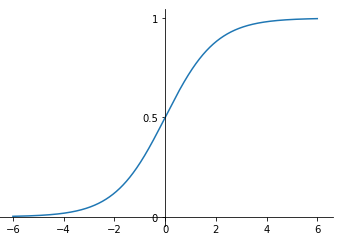一般情况在z值是较难确定的，所以引入梯度下降算法，进行线性回归系数的拟合计算。
3.梯度下降算法
梯度下降原理在机器学习和模型优化中有着十分重要的作用。类似于：将一个球从山顶往山脚滚动，先加速，到一定时候减速。与sigmoid函数图像相类似，有的时候也会用sigmoid函数作为梯度下降函数。
线性回归函数公式：

其中θ为线性回归系数,梯度下降用来求解回归系数.
损失函数公式:

更新权重，对损失函数求偏导数:

演变为：

二、实例
以研究生录取为例，采取字段成绩，GPA和排名字段，判断该研究生是否录取。
样本数据如下：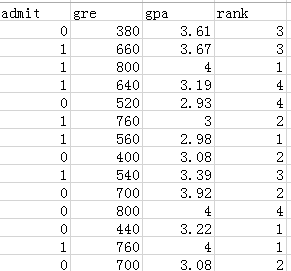实现录取判别的原理：
构造逻辑回归函数——》梯度下降，计算一元多项式回归系数——》更新逻辑回归函数——》计算预测结果

逻辑回归函数：
为了提高样本数据的线性相关性，将y=0的值映射到【-6,0)区间范围内，y=1的值映射到[0,6]区间范围内。那么对应逻辑回归计算的y=0时的概率<0.5；y=1的概率>=0.5
观察样本数据，发现‘成绩’（gre）字段的数据较其他的数据大，所以对该字段进行标准化处理。本次标准化采用z-score 标准化
公式：新数据 =（原数据 - 均值）/标准差
下面就是编写程序代码了，所有的代码都是封装成模块运行的：
逻辑回归模型构建：
文件：logisticReression.py
from numpy import *

class Logistic_Reression():

def __init__(self, train_data, y, test_data): #将y(0,1)处理成(0,6)

self.train_data = train_data #数据维度，一元多项式的项
self.test_data = test_data
self.y = y

def sigmoid(self,x):

return 1./(1+exp(-x))

def linear_regression(self, x, coeDict): #线性回归函数

formula = coeDict

for i in range(1,len(coeDict)):

formula += coeDict[i]*x[i-1]

return formula

def Get_coefficient(self): #梯度下降函数，用来得到回归系数

#数据维度
k = self.train_data.shape #需要计算的次数
y_train = self.train_y()
#初始化参数
coeDict = random.normal(size=k+1)
coeList = *(k+1)

for n in range(1000):

for x,y in zip(self.train_data, y_train):

y1 = self.linear_regression(x, coeDict)
coeList += 0.001*(y - y1)

for i in range(1,k+1):
rate = 0.0001# + 4/(i+n)
coeList[i] += rate*(y - y1)*x[i-1]

if (inf in coeList) | (-inf in coeList): break

return coeDict

def train_y(self):#处理y的值,使他具有线性相关性

y = random.uniform(-6, 0, size=len(self.y)) #随机生成大于[-6,0)的数

for i in range(len(self.y)):

if (self.y[i] == 1): y[i] = random.uniform(0, 7) #y[i] = 6

y.sort()

return y

def logistic_reression(self): #计算模型预测值,返回值为[0,1]的概率

coeDict = self.Get_coefficient()
y = self.train_y()

forecast_y = []
formula = *len(self.train_data)

for i in range(len(self.train_data)):

formula[i] = self.linear_regression(self.train_data[i],coeDict)
result = self.sigmoid(formula[i])

if (result >= 0.5): result = 1
else: result = 0
forecast_y.append(result)

return forecast_y

模型评价指标
文件：evaluationIndex.py
from numpy import *
import pandas as pd
import seaborn as sns
import matplotlib.pyplot as plt
from pylab import mpl

#%matplotlib inline
sns.set()
#修改字体，解决中文乱码
mpl.rcParams['font.sans-serif'] = ['FangSong']
mpl.rcParams['axes.unicode_minus'] = False

class Evaluation_Index(): #模型评价指标

def __init__(self, realData, forecastData):

self.realData = realData
self.forecastData = forecastData

def MSE(self): #均方误差

return sum((self.realData - self.forecastData)**2)/len(self.realData)

def Accuracy(self): #准确率

df = pd.DataFrame({'ID':list(range(1,len(self.realData)+1)), 'real_data':self.realData,
'forecast_data':self.forecastData})      #分类结果组成矩阵
Acc = len(df[df.real_data == df.forecast_data])/360       #分类精确度,确认输入的是判别模型的值。应该为0,1

return Acc

def ConfusionMatrix(self): #混淆矩阵

df = pd.DataFrame({'ID':list(range(1,len(self.realData)+1)), 'real_data':self.realData,
'forecast_data':self.forecastData})      #分类结果组成矩阵
Acc = len(df[df.real_data == df.forecast_data])       #分类精确度,确认输入的是判别模型的值。应该为0,1
Confuse_matrix = df.groupby(['real_data','forecast_data']).count() #混淆矩阵
Confuse_matrix = Confuse_matrix.unstack()
Confuse_matrix = Confuse_matrix.fillna(0).astype(int)
#绘制混淆矩阵图像
sns.heatmap(Confuse_matrix.T, square=True, annot=True, fmt='d', cbar=False,
xticklabels=Confuse_matrix.index, yticklabels=Confuse_matrix.columns.labels)
plt.xlabel('真实数据')
plt.ylabel('预测数据')

return Confuse_matrix  
主程序：main.py文件
import pandas as pd
from numpy import *
import importlib
import evaluationIndex
import logisticReression

def scale(data): #标标准化公式

return (data - mean(data))/std(data)

def handingData(dateframe): #数据处理

#标准化处理
dateframe.gre = scale(data=dateframe)
dateframe.gpa = scale(data=dateframe)

#抽取训练集和检验集
trainData = dateframe.sample(frac=0.9)
testData = dateframe.sample(frac=0.1)

#数据处理，使之具有线性回归性质
trainData = trainData.sort_values(by=['admit', 'rank', 'gre', 'gpa'], ascending=(True, False, True, True))
trainData.reindex(range(len(trainData)))
testData = testData.sort_values(by=['admit', 'rank', 'gre', 'gpa'], ascending=(True, False, True, True))
testData.reindex(range(len(testData)))

train_data = array(trainData.loc[:, 'gre':'rank'])
test_data = array(testData.loc[:, 'gre':'rank'])

return train_data, train_y, test_data, realData

if __name__ == '__main__':

data_route = r'E:/data/logic_res/luqu2.xlsx' #数据路径

#print(df)
train_data, train_y, test_data, realData = handingData(df)
model = logisticReression.Logistic_Reression(train_data=train_data, y=train_y)
forecast_data = model.logistic_reression()

Index = evaluationIndex.Evaluation_Index(train_y, forecast_data)
acc = Index.Accuracy()
Confuse_matrix = Index.ConfusionMatrix()
print('准确率：',round(acc,4))
print('混淆矩阵：\n', Confuse_matrix)
运行结果：准确率为0.6972，混淆矩阵如下图：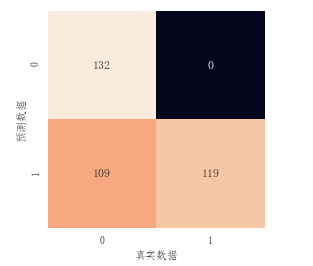混淆矩阵显示结果中：0类判断全部正确，1类的判断为0的误差较大。考虑到数据本身的质量问题了或者真实性问题。并且由于回归系数的初始值是随机生成，初始回归系数的不同导致模型预测的准确率不一致。所以小编就想尽可能的去拟合模型，测试该模型最大的准确率能达到多少。
代码也很简单，写个循环就够了，将执行语句修改为：
if __name__ == "__main__":

data_route = r'E:/data/logic_res/luqu2.xlsx' #数据路径

#print(df)
train_data, train_y, test_data, realData = handingData(df)

Acc = 0
function = None
#由于回归系数的初始值不同，导致每一次计算的准确率不一致。所以循环运行该程序，计算准确率最高的值
for i in range(50):

#调用模型
model = logisticReression.Logistic_Reression(train_data=train_data, y=train_y)
forecast_data = model.logistic_reression()

Index = evaluationIndex.Evaluation_Index(train_y, forecast_data)
acc = Index.Accuracy()

if (acc > Acc):  Acc = acc; function = Index
if round(Acc,4) >= 0.9999: break

Confuse_matrix = function.ConfusionMatrix()
print('准确率：',round(Acc,4))
print('混淆矩阵：\n', Confuse_matrix)
循环50次后，模型的准确率居然能达到1.0，也就是完全拟合。这肯定是不可能的。然后我们以该模型去预测检验集。
修改logisticReression.py文件中logistic_reression()函数部分代码：
for i in range(len(self.test_data)):

formula[i] = self.linear_regression(self.test_data[i],coeDict)
模型的准确率为0.1，说明之前的模型出现了过拟合现象。
一般在做线性回归的时候，我们都会考虑到模型的可解释性，如果模型解释性较差，则认为模型的预测效果较差。
这一般都是在数据建模之前应该考虑的问题。由于该样本数据在大部分学习视频中和网上都有，所以小编也没有怀疑该样本数据是否能进行逻辑回归模型的建模。这也是一大坑。
直接调用Python statsmodels.formula.api 模块中的ols函数进行了简单粗暴的观察，发现模型的R方不到0.1，所以对其进行标准化处理和提高线性相关性的处理。
代码如下：
from statsmodels.formula.api import ols

data_route = r'E:/data/logic_res/luqu2.xlsx' #数据路径

# df.gre = (df.gre - mean(df.gre))/std(df.gre)
# df.gpa = (df.gpa - mean(df.gpa))/std(df.gpa)
df = df.sort_values(by=['admit', 'rank', 'gre', 'gpa'], ascending=(True, False, True, True))
df.reindex(range(len(df)))

y1 = random.uniform(-6, 0, size=len(df[df.admit == 0])) #随机生成大于[-6,0)的整数
y2 = random.uniform(0, 7, size=len(df[df.admit == 1]))
y = hstack((y1,y2))
df['y'] = sort(y)
mld = ols('y~gre+gpa+rank', df).fit()
print(mld.summary())
结果如下：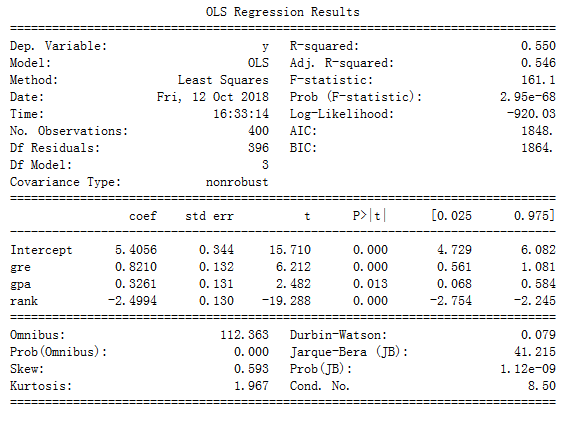该函数可以计算出很多指标，主要观察 R-squared即为0.550，较标准化处理和线性回归处理前有明显的提高，但也较低。也就是说该模型的可解释性较低。
也可以计算参数之间的相关系数。使用numpy下的corrcoef函数。代码如下：
cor = corrcoef(df.iloc[:, :4], rowvar=0)
names = df.iloc[:, :4].columns
cor = pd.DataFrame(cor, index=names, columns=names)
print(cor)
结果：
各指标之间的相关系数都不高。在假设数据真实可靠地前提下，认为仅仅凭借成绩、GPA和排名，不能确定是否通过考研。
展开全文梯度下降
• 最终用户只需输入很少的内容，就可以分别处理数字，分类和日期时间功能。 这听起来不令人兴奋吗？ REGML-回归ML 该应用程序旨在帮助数据科学家分析回归数据集并推荐最佳ML模型。 数据应以csv / txt格式提供，并且...
• f^(x)=fM(x)=∑m=1M∑j=1JcmjI(x∈Rmj)\hat{f}(x)=f_M(x)=\sum_{m=1}^{M}\sum_{j=1}^{J}c_{mj}I(x\in R_{mj})f^​(x)=fM​(x)=∑m=1M​∑j=1J​cmj​I(x∈Rmj​) GBDT分类算法 从思想上看，GBDT分类算法和回归算法...
GBDT
在前几年的机器学习竞赛以及工作中，人们使用着各种传统算法进行调参取得性能的提升，突然有一天杀出了一种名为GBDT的算法，改变了当前的格局，该算法在不同的场景中总是能够产生很好的效果，本文就让我们来了解一下GBDT。
DT：CART回归树
这里需要注意的是我们使用的决策树是CART回归树，无论是我们要处理回归问题还是分类问题，我们都需要使用CART回归树，主要是因为我们的GBDT算法每次迭代要拟合的是梯度值，这是一个连续值，因此我们需要使用CART回归树。
对于回归树算法来说最重要的是寻找最佳的划分点，那么回归树中的可划分点包含了所有特征的所有可取的值。在分类树中最佳划分点的判别标准是熵或者基尼系数，都是用纯度来衡量的，但是在回归树中的样本标签是连续数值，所以再使用熵之类的指标不再合适，取而代之的是平方误差，它能很好的评判拟合程度。
关于CART回归树的生成方式具体可以参考我们之前的文章：
GB：梯度提升
我们本文中提到的GBDT其实是提升树（Boosting Tree）的一种改进算法，我们先来看一下BT是怎样进行工作的。
Boosting Tree的流程
（1）初始化

f

0

(

x

)

=

0

f_0(x)=0

（2）对m=1,2,…,M
​ （a）计算残差
​

r

m

i

=

y

i

−

f

m

−

1

(

x

i

)

,

i

=

1

,

2

,

.

.

.

,

N

r_{mi}=y_i-f_{m-1}(x_i),i=1,2,...,N

​ （b）拟合残差

r

m

i

r_{mi}

学习一个回归树，得到

h

m

(

x

)

h_m(x)

​ （c）更新

f

m

(

x

)

=

f

m

−

1

+

h

m

(

x

)

f_m(x)=f_{m-1}+h_m(x)

（3）得到回归问题提升树

f

M

(

x

)

=

∑

m

=

1

M

h

m

(

x

)

f_M(x)=\sum_{m=1}^Mh_m(x)

对于上面的流程中残差又代表着什么呢？
假设我们前一轮迭代得到的强学习器是：

f

t

−

1

(

x

)

f_{t-1}(x)

损失函数是：

L

(

y

,

f

t

−

1

(

x

)

)

L(y,f_{t-1}(x))

我们本轮迭代的目标是找到一个弱学习器：

h

t

(

x

)

h_t(x)

使得本轮的损失函数最小化：

L

(

y

,

f

t

(

x

)

)

=

L

(

y

,

f

t

−

1

(

x

)

+

h

t

(

x

)

)

L(y,f_t(x))=L(y,f_{t-1}(x)+h_t(x))

此时的损失采用平方损失函数时：

L

(

y

,

f

t

−

1

(

x

)

+

h

t

(

x

)

)

L(y,f_{t-1}(x)+h_t(x))

=

(

y

−

f

t

−

1

(

x

)

−

h

t

(

x

)

)

2

=(y-f_{t-1}(x)-h_t(x))^2

=

(

r

−

h

t

(

x

)

)

2

=(r-h_t(x))^2

此时有残差：

r

=

y

−

f

t

−

1

(

x

)

r=y-f_{t-1}(x)

举个例子来表示一下残差：
假设小明有100元，第一次我们用80元去拟合，此时的残差就是20元，第二次我们用10元去拟合剩下的残差（损失：即20元），此时的残差是10元，依次类推我们每一次都去拟合剩下的残差，对于提升树来说我们把每一次拟合的钱数加起来就是模型最终的输出结果了。
负梯度的表达形式如下：

−

[

∂

L

(

y

,

f

(

x

i

)

)

∂

f

(

x

i

)

]

f

(

x

)

=

f

m

−

1

(

x

)

-[\frac{\partial L(y,f(x_i))}{\partial f(x_i)}]_{f(x)=f_{m-1}(x)}

GBDT回归算法的流程
输入：训练数据集

T

=

{

(

x

1

,

y

1

)

,

(

x

2

,

y

2

)

,

.

.

.

,

(

x

N

,

y

N

)

}

T=\{(x_1,y_1),(x_2,y_2),...,(x_N,y_N)\}

，

x

i

∈

X

⊆

R

n

x_i\in X \subseteq R^n

，

y

i

∈

Y

⊆

R

y_i\in Y \subseteq R

；损失函数

L

(

y

,

f

(

x

)

)

L(y,f(x))

；
输出：回归树

f

^

(

x

)

\hat{f}(x)

（1）初始化弱学习器：

f

0

(

x

)

=

a

r

g

m

i

n

c

∑

i

=

1

N

L

(

y

i

,

c

)

f_0(x)=arg\underset{c}{min}\sum_{i=1}^NL(y_i,c)

（2）对m=1,2,…,M
​ （a）对i=1,2,…,N，计算负梯度（残差）：

r

m

i

=

−

[

∂

L

(

y

,

f

(

x

i

)

)

∂

f

(

x

i

)

]

f

(

x

)

=

f

m

−

1

(

x

)

r_{mi}=-[\frac{\partial L(y,f(x_i))}{\partial f(x_i)}]_{f(x)=f_{m-1}(x)}

​ （b）将a中得到的残差作为新样本的真实值，拟合一个回归树，得到第m棵树的叶子结点区域

R

m

j

R_{mj}

，

j

=

1

,

2

,

.

.

.

,

J

j=1,2,...,J

。其中

J

J

为回归树的叶子结点的个数。
​ （c）对

j

=

1

,

2

,

.

.

.

,

J

j=1,2,...,J

，计算最佳拟合值：

c

m

j

=

a

r

g

m

i

n

c

∑

x

i

∈

R

m

j

L

(

y

i

,

f

m

−

1

(

x

i

)

+

c

)

c_{mj}=arg\underset{c}{min}\sum_{x_i\in R_{mj}}L(y_i,f_{m-1}(x_i)+c)

​ （d）更新强学习器

f

m

(

x

)

=

f

m

−

1

(

x

)

+

∑

j

=

1

J

c

m

j

I

(

x

∈

R

m

j

)

f_m(x)=f_{m-1}(x)+\sum_{j=1}^Jc_{mj}I(x\in R_{mj})

（I为指示函数）
（3）得到最终的学习器

f

^

(

x

)

=

f

M

(

x

)

=

∑

m

=

1

M

∑

j

=

1

J

c

m

j

I

(

x

∈

R

m

j

)

\hat{f}(x)=f_M(x)=\sum_{m=1}^{M}\sum_{j=1}^{J}c_{mj}I(x\in R_{mj})

GBDT分类算法
从思想上看，GBDT分类算法和回归算法没有区别，但是对于分类来说我们的样本输出不是连续的值，而是离散的类别，这也导致我们无法直接从输出类别去拟合类别输出的误差。
GBDT二分类算法
我们使用类似于逻辑回归的对数似然函数时，损失函数为：

L

(

y

,

f

(

x

)

)

=

l

o

g

(

1

+

e

x

p

(

−

y

f

(

x

)

)

)

L(y,f(x))=log(1+exp(-yf(x)))

，

y

∈

{

−

1

,

+

1

}

y\in\{-1,+1\}

则此时的负梯度误差为：

r

m

i

=

−

[

∂

L

(

y

,

f

(

x

i

)

)

∂

f

(

x

i

)

]

f

(

x

)

=

f

m

−

1

(

x

)

=

y

i

(

1

+

e

x

p

(

y

i

f

(

x

i

)

)

)

r_{mi}=-[\frac{\partial L(y,f(x_i))}{\partial f(x_i)}]_{f(x)=f_{m-1}(x)}=\frac{y_i}{(1+exp(y_if(x_i)))}

对于生成的决策树，我们各个叶子结点的最佳负梯度拟合值为：

c

m

j

=

a

r

g

m

i

n

c

∑

x

i

∈

R

m

j

l

o

g

(

1

+

e

x

p

(

−

y

i

(

f

m

−

1

(

x

i

)

+

c

)

)

)

c_{mj}=arg\underset{c}{min}\sum_{x_i\in R_{mj}}log(1+exp(-y_i(f_{m-1}(x_i)+c)))

由于上式难以优化，我们一般使用近似值代替：

c

m

j

=

∑

x

i

∈

R

m

j

r

m

i

∑

x

i

∈

R

m

j

∣

r

m

i

∣

(

1

−

∣

r

m

i

∣

)

c_{mj}=\frac{\sum_{x_i\in R_{mj}}r_{mi}}{\sum_{x_i\in R_{mj}}|r_{mi}|(1-|r_{mi}|)}

出了负梯度计算和叶子结点的最佳负梯度拟合的线性搜索，GBDT二元分类和GBDT回归算法过程相同。
GBDT多分类算法
假设类别为K，则此时我们的对数似然损失函数为：

L

(

y

,

f

(

x

)

)

=

−

∑

k

=

1

k

y

k

l

o

g

(

p

k

(

x

)

)

L(y,f(x))=-\sum_{k=1}^ky_klog(p_k(x))

其中如果样本输出类别为k，则

y

k

=

1

y_k=1

。第k类的概率

p

k

(

x

)

p_k(x)

的表达式为：

p

k

(

x

)

=

e

x

p

(

f

k

(

x

)

)

∑

l

=

1

k

e

x

p

(

f

l

(

x

)

)

p_k(x)=\frac{exp(f_k(x))}{\sum_{l=1}^kexp(f_l(x))}

集合上两式，我们可以计算出第t轮的第i个样本对应类别l的负梯度误差为：

r

m

i

l

=

−

[

∂

L

(

y

,

f

(

x

i

)

)

∂

f

(

x

i

)

]

f

(

x

)

=

f

l

,

m

−

1

(

x

)

=

y

i

l

−

p

l

,

m

−

1

(

x

i

)

r_{mil}=-[\frac{\partial L(y,f(x_i))}{\partial f(x_i)}]_{f(x)=f_{l,m-1}(x)}=y_{il}-p_{l,m-1}(x_i)

根据上式我们就能够知道，这里得到的负梯度的误差其实就是真实概率和t-1轮预测概率的差值。
对于生成的决策树，我们各个叶子结点的最佳负梯度拟合值为：

c

m

j

l

=

a

r

g

m

i

n

c

j

l

∑

i

=

0

m

∑

k

=

1

k

L

(

y

k

,

f

m

−

1

,

l

(

x

)

+

∑

j

=

1

J

c

j

l

I

(

x

i

∈

R

m

j

l

)

)

c_{mjl}=arg\underset{c_{jl}}{min}\sum_{i=0}^{m}\sum_{k=1}^kL(y_k,f_{m-1,l}(x)+\sum_{j=1}^{J}c_{jl}I(x_i\in R_{mjl}))

同理我们使用近似值代替：

c

m

j

l

=

K

−

1

K

∑

x

i

∈

R

m

j

l

r

m

i

l

∑

x

i

∈

R

m

j

l

∣

r

m

i

l

∣

(

1

−

∣

r

m

i

l

∣

)

c_{mjl}=\frac{K-1}{K}\frac{\sum_{x_i\in R_{mjl}}r_{mil}}{\sum_{x_i\in R_{mjl}}|r_{mil}|(1-|r_{mil}|)}

除了负梯度计算和叶子节点的最佳负梯度拟合的线性搜索，GBDT多分类和GBDT二分类以及GBDT回归算法过程相同。
GBDT的过拟合
为了防止过拟合问题的产生，我们也需要对GBDT采取一些措施，主要的方式有以下的三种：
剪枝
剪枝是树模型中常用的防止过拟合的方法，在GBDT中同样适用。
定义步长
我们把步长定义为v，对于前面的弱学习器的迭代：

f

k

(

x

)

=

f

k

−

1

(

x

)

+

h

k

(

x

)

f_k(x)=f_{k-1}(x)+h_k(x)

如果我们加上了正则化项，则有：

f

k

(

x

)

=

f

k

−

1

(

x

)

+

v

h

k

(

x

)

f_k(x)=f_{k-1}(x)+vh_k(x)

v的取值范围为(0,1]，对于同样的训练集学习效果，较小的v意味着我们需要更多的弱学习器的迭代次数，通常我们用步长和迭代最大次数一起来决定算法的拟合效果。
子采样比例
我们还可以通过子采样比例的方式来防止过拟合，取值为(0,1]，这里的子采样和随机森林不一样，随机森林使用的是放回抽样，而这里是不放回抽样。如果取值为1，则全部样本都使用，等于没有使用子采样。如果取值小于1，则只有一部分样本会去做GBDT的决策树拟合。选择小于1的比例可以减少方差，即防止过拟合，但是会增加样本拟合的偏差，因此取值不能太低。推荐在[0.5, 0.8]之间。
GBDT的优缺点
优点
预测精度高；适合低维数据；能处理非线性数据；可以灵活处理各种类型的数据，包括连续值和离散值；在相对少的调参时间情况下，预测的准备率也可以比较高。这个是相对SVM来说的；使用一些健壮的损失函数，对异常值的鲁棒性非常强。比如 Huber损失函数和Quantile损失函数；
缺点
由于弱学习器之间存在依赖关系，难以并行训练数据。不过可以通过自采样的SGBT来达到部分并行；如果数据维度较高时会加大算法的计算复杂度。展开全文算法 决策树 机器学习 人工智能 GBDT
• pytorch一维线性回归程序pytorch一维线性回归程序pytorch一维线性回归程序pytorch
• * cox比例风险回归模型其R程序教学课件 * ti 时刻的基础生存率公式 ti 时刻的基础累计风险函数公式 在tk时刻的死亡人数 ti 时刻的生存率计算公式 2.Breslow法 * cox比例风险回归模型其R程序教学课件 * 5....
• 1.使用Logistic回归梯度上升优化算法  每次更新回归系数都要遍历整个数据集，该算法在处理100左右各样本时还可以，但是如果有数十亿样本或者成千上万的特征，那么该算法就太过于复杂了。 import os from numpy ...
• 这里用到的数据是Andrew老师在coursera授课时作业中的数据，由于上传过程中公式格式有点乱，因此省掉了原理部分，具体的可以看Andrew老师的授课视频讲义。 两分类问题，特征值为学生的两门课考试成绩，y值0、...机器学习
• 程序是用python代码实现的逻辑回归，里面有详细的注释，代码量不多，请放心参考。
• 亚马逊食物评论二分类，使用的是线性回归模型。。正负样本近20万条。。。模型已经训练好了，有94%的正确率。导入eclipse即可运行tensorflow
• matlab开发-分类逻辑回归。二类多类分类的Logistic回归
• 随机森林模型有着惊人的准确性，可以替代一般线性模型（线性回归、方差分析等）广义线性模型（逻辑斯蒂回归、泊松回归等）等等。   我2012年在人民大学组织的R语言会议上介绍了随机森林的用法（报告文件在...数字图像处理
• 包括能够进行多变量估计降维的回归变量，以及基于回归到单变量多变量表示的单变量分类器。 此存储库还旨在作为一个精简示例，说明如何使用TravisCI，Coveralls，Sphinx，PyTest，如何部署到PyPIGithub Pages，...
• 逻辑回归知识要点一、逻辑回归模型模型简介：算法的分类思想算法模型sigmoid函数函数原型sigmoid函数图像参数求解二、逻辑回归实现二分类模型训练与预测结果可视化计算概率值绘制决策边界三、逻辑回归实现多分类建模...python 机器学习
• 我们将逻辑回归和DTRS集成在一起，以提供一种新的分类方法。一方面，DTRS用于通过贝叶斯决策程序系统地计算相应的阈值。另一方面，采用逻辑回归来计算三向决策的条件概率。企业失败预测高中课程选择的预测的实证...
•机器学习
• 逻辑回归是预测分类响应的常用方法。广义线性模型的一个特例是预测结果的概率。在spark.ml逻辑回归中，可以使用二项Logistic回归来预测二元结果，或者可以使用多项Logistic回归来预测多类结果。使用该family 参数在...MLlib 算法 机器学习
• logistic回归分类是一种简单的分类算法。在分类的过程中只需要找到一个划分不同类的权重向量即可，对新的数据只需要乘上这个向量并比较就可以得到分类。比如下图的二分类问题： 每个样本点可以看成包含两个特征...机器学习 数据
• %本程序读取training-a中150个心音信号作为训练集，后150个心音信号作为测试数据，采用SVM支持向量机逻辑回归分类器 %进行心音信号的分类 %% clear; clc; rng=[0 1 149 1];%训练 rng=[r1 c1 r2 c2]定义读取csv文件...svm
• elm回归分类：ELM是一种简单易用、有效的单隐层前馈神经网络SLFNs学习算法。2004年由南洋理工大学黄广斌副教授提出。传统的神经网络学习算法（如BP算法）需要人为设置大量的网络训练参数，并且很容易产生局部最优...elm
• 首先给大家强调一点，这是一个分类模型而不是一个回归模型！下文开始将从不同方面讲解logistic回归的原理，随后分别使用梯度上升算法随机梯度上升算法将logistic回归算法应用到实例中。 一、logistic回归和线性...
• ## 分类，聚类及其回归的区别

万次阅读 多人点赞 2018-05-06 19:38:06
from：...由上图我们可以看到，机器学习分为四大块，分别是classification (分类)，regression (回归),clustering (聚类),dimensionality reduction (降维...聚类 数据挖掘
• ML之分类预测之LARS：利用回归工具将二分类转为回归问题并采用LARS算法构建分类器 输出结果 ['V10', 'V48', 'V44', 'V11', 'V35', 'V51', 'V20', 'V3', 'V21', 'V15', 'V43', 'V0', 'V22', 'V45', 'V53', 'V27'......